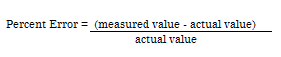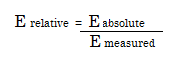# Measurement Error (Observational Error)

Share on

Bias > Measurement Error

## What is Measurement Error?

Measurement Error (also called Observational Error) is the difference between a measured quantity and its true value. It includes random error (naturally occurring errors that are to be expected with any experiment) and systematic error (caused by a mis-calibrated instrument that affects all measurements).

For example, let’s say you were measuring the weights of 100 marathon athletes. The scale you use is one pound off: this is a systematic error that will result in all athletes body weight calculations to be off by a pound. On the other hand, let’s say your scale was accurate. Some athletes might be more dehydrated than others. Some might have wetter (and therefore heavier) clothing or a 2 oz. candy bar in a pocket. These are random errors and are to be expected. In fact, all collected samples will have random errors — they are, for the most part, unavoidable.

Measurement errors can quickly grow in size when used in formulas. For example, if you’re using a small error in a velocity measurement to calculate kinetic energy, your errors can easily quadruple. To account for this, you should use a formula for error propagation whenever you use uncertain measures in an experiment to calculate something else.

## Different Measures of Error

Different measures of error include:

1. Absolute Error: the amount of error in your measurement. For example, if you step on a scale and it says 150 pounds but you know your true weight is 145 pounds, then the scale has an absolute error of 150 lbs – 145 lbs = 5 lbs.
2. Greatest Possible Error: defined as one half of the measuring unit. For example, if you use a ruler that measures in whole yards (i.e. without any fractions), then the greatest possible error is one half yard.
3. Instrument Error: error caused by an inaccurate instrument (like a scale that is off or a poorly worded questionnaire).
4. Margin of Error: an amount above and below your measurement. For example, you might say that the average baby weighs 8 pounds with a margin of error of 2 pounds (± 2 lbs).
5. Measurement Location Error: caused by an instrument being placed somewhere it shouldn’t, like a thermometer left out in the full sun.
6. Operator Error: human factors that cause error, like reading a scale incorrectly.
7. Percent Error: another way of expressing measurement error. Defined as:8. Relative Error: the ratio of the absolute error to the accepted measurement. As a formula, that’s:## Ways to Reduce Measurement Error

• Double check all measurements for accuracy. For example, double-enter all inputs on two worksheets and compare them.
• Double check your formulas are correct.
• Make sure observers and measurement takers are well trained.
• Make the measurement with the instrument that has the highest precision.
• Take the measurements under controlled conditions.
• Pilot test your measuring instruments. For example, put together a focus group and ask how easy or difficult the questions were to understand.
• Use multiple measures for the same construct. For example, if you are testing for depression, use two different questionnaires.

## Statistical Procedures to Assess Measurement Error

The following methods assess “absolute reliability”:

• Standard error of measurement (SEM): estimates how repeated measurements taken on the same instrument are estimated around the true score.
• Coefficient of variation (CV): a measure of the variability of a distribution of repeated scores or measurements. Smaller values indicate a smaller variation and therefore values closer to the true score.
• Limits of agreement (LOA): gives an estimate of the interval where a proportion of the differences lie between measurements.

## References

Beyer, W. H. CRC Standard Mathematical Tables, 31st ed. Boca Raton, FL: CRC Press, pp. 536 and 571, 2002.
Dodge, Y. (2008). The Concise Encyclopedia of Statistics. Springer.
Vogt, W.P. (2005). Dictionary of Statistics & Methodology: A Nontechnical Guide for the Social Sciences. SAGE.
Wheelan, C. (2014). Naked Statistics. W. W. Norton & Company

CITE THIS AS:
Stephanie Glen. "Measurement Error (Observational Error)" From StatisticsHowTo.com: Elementary Statistics for the rest of us! https://www.statisticshowto.com/measurement-error/
---------------------------------------------------------------------------Need help with a homework or test question? With Chegg Study, you can get step-by-step solutions to your questions from an expert in the field. Your first 30 minutes with a Chegg tutor is free!